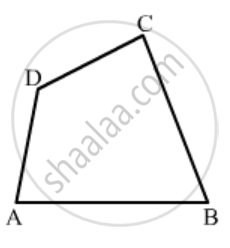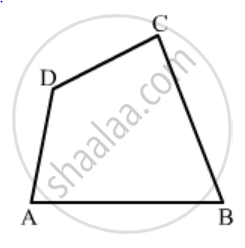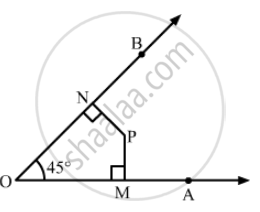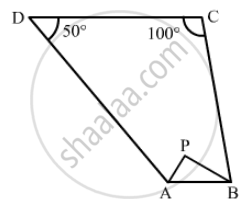Share

# RD Sharma solutions for Class 8 Mathematics chapter 16 - Understanding Shapes-II (Quadrilaterals)

#### RD Sharma Mathematics Class 8 by R D Sharma (2019-2020 Session)## Chapter 16: Understanding Shapes-II (Quadrilaterals)

Ex. 16.10

#### Chapter 16: Understanding Shapes-II (Quadrilaterals) Exercise 16.10 solutions [Pages 15 - 17]

Ex. 16.10 | Q 1.1 | Page 15

Define the following term Quadrilateral .

Ex. 16.10 | Q 1.2 | Page 15

Define the following term Convex Quadrilateral .

Ex. 16.10 | Q 2.01 | Page 15

In a quadrilateral, define of the following Sides.

Ex. 16.10 | Q 2.02 | Page 15

In a quadrilateral, define of the following   Vertices .

Ex. 16.10 | Q 2.03 | Page 15

In a quadrilateral, define of the following  Angles .

Ex. 16.10 | Q 2.04 | Page 15

In a quadrilateral, define of the following Diagonals .

Ex. 16.10 | Q 2.05 | Page 15

Ex. 16.10 | Q 2.06 | Page 15

Ex. 16.10 | Q 2.07 | Page 15

In a quadrilateral, define of the following Opposite sides .

Ex. 16.10 | Q 2.08 | Page 15

In a quadrilateral, define of the following  Opposite angles .

Ex. 16.10 | Q 2.09 | Page 15

In a quadrilateral, define of the following Interior .

Ex. 16.10 | Q 2.1 | Page 15

In a quadrilateral, define of the following  Exterior .

Ex. 16.10 | Q 3.01 | Page 15

Complete of the following, so as to make a true statement:

Ex. 16.10 | Q 3.02 | Page 15

Complete of the following, so as to make a true statement:

Ex. 16.10 | Q 3.03 | Page 15

Complete of the following, so as to make a true statement:

A quadrilateral has ..... vertices, no three of which are .....

Ex. 16.10 | Q 3.04 | Page 15

Complete of the following, so as to make a true statement:

Ex. 16.10 | Q 3.05 | Page 15

Complete of the following, so as to make a true statement:

The number of pairs of adjacent angles of a quadrilateral is .......

Ex. 16.10 | Q 3.06 | Page 15

Complete of the following, so as to make a true statement:

The number of pairs of opposite angles of a quadrilateral is .......

Ex. 16.10 | Q 3.07 | Page 15

Complete of the following, so as to make a true statement:

The sum of the angles of a quadrilateral is ......

Ex. 16.10 | Q 3.08 | Page 15

Complete of the following, so as to make a true statement:

A diagonal of a quadrilateral is a line segment that joins two ...... vertices of the quadrilateral.

Ex. 16.10 | Q 3.09 | Page 15

Complete of the following, so as to make a true statement:

The sum of the angles of a quiadrilateral is .... right angles.

Ex. 16.10 | Q 3.1 | Page 15

Complete of the following, so as to make a true statement:

The measure of each angle of a convex quadrilateral is ..... 180°.

Ex. 16.10 | Q 3.11 | Page 15

Complete of the following, so as to make a true statement:

In a quadrilateral the point of intersection of the diagonals lies in .... of the quadrilateral.

Ex. 16.10 | Q 3.12 | Page 15

Complete of the following, so as to make a true statement:

A point is in the interior of a convex quadrilateral, if it is in the ..... of its two opposite angles.

Ex. 16.10 | Q 3.13 | Page 15

Complete of the following, so as to make a true statement:

A quadrilateral is convex if for each side, the remaining .... lie on the same side of the line containing the side.

Ex. 16.10 | Q 4.1 | Page 16

In Fig. 16.19, ABCD is a quadrilateral.

Name a pair of adjacent sides.Ex. 16.10 | Q 4.2 | Page 16

In Fig. 16.19, ABCD is a quadrilateral.

Name a pair of opposite sides.Ex. 16.10 | Q 4.3 | Page 16

In Fig. 16.19, ABCD is a quadrilateral.

How many pairs of adjacent sides are there?Ex. 16.10 | Q 4.4 | Page 16

In Fig. 16.19, ABCD is a quadrilateral.

How many pairs of opposite sides are there?Ex. 16.10 | Q 4.5 | Page 16

In Fig. 16.19, ABCD is a quadrilateral.

Name a pair of adjacent angles.Ex. 16.10 | Q 4.6 | Page 16

In Fig. 16.19, ABCD is a quadrilateral.

Name a pair of opposite angles.Ex. 16.10 | Q 4.7 | Page 16

In Fig. 16.19, ABCD is a quadrilateral.

How many pairs of adjacent angles are there?Ex. 16.10 | Q 4.8 | Page 16

In Fig. 16.19, ABCD is a quadrilateral.

How many pairs of opposite angles are there?Ex. 16.10 | Q 5 | Page 16

The angles of a quadrilateral are 110°, 72°, 55° and x°. Find the value of x.

Ex. 16.10 | Q 6 | Page 16

The three angles of a quadrilateral are respectively equal to 110°, 50° and 40°. Find its fourth angle.

Ex. 16.10 | Q 7 | Page 16

A quadrilateral has three acute angles each measures 80°. What is the measure of the fourth angle?

Ex. 16.10 | Q 8 | Page 16

A quadrilateral has all its four angles of the same measure. What is the measure of each?

Ex. 16.10 | Q 9 | Page 16

Two angles of a quadrilateral are of measure 65° and the other two angles are equal. What is the measure of each of these two angles?

Ex. 16.10 | Q 10 | Page 16

Three angles of a quadrilateral are equal. Fourth angle is of measure 150°. What is the measure of equal angles.

Ex. 16.10 | Q 11 | Page 16

The four angles of a quadrilateral are as 3 : 5 : 7 : 9. Find the angles.

Ex. 16.10 | Q 12 | Page 16

If the sum of the two angles of a quadrilateral is 180°. What is the sum of the remaining two angles?

Ex. 16.10 | Q 13 | Page 16

In Fig. 16.20, find the measure of ∠MPNEx. 16.10 | Q 14 | Page 16

The sides of a quadrilateral are produced in order. What is the sum of the four exterior angles?

Ex. 16.10 | Q 15 | Page 16

In Fig. 16.21, the bisectors of ∠A and ∠B meet at a point P. If ∠C = 100° and ∠D = 50°, find the measure of ∠APBEx. 16.10 | Q 16 | Page 17

In a quadrilateral ABCD, the angles A, B, C and D are in the ratio 1 : 2 : 4 : 5. Find the measure of each angle of the quadrilateral.

Ex. 16.10 | Q 17 | Page 17

In a quadrilateral ABCD, CO and DO are the bisectors of ∠C and ∠D respectively. Prove that $∠COD = \frac{1}{2}(∠A + ∠B) .$

Ex. 16.10 | Q 18.1 | Page 17

Find the number of side of a regular polygon, when of its angle has a measure of 160° .

Ex. 16.10 | Q 18.2 | Page 17

Find the number of side of a regular polygon, when of its angle has a measure of 135° .

Ex. 16.10 | Q 18.3 | Page 17

Find the number of side of a regular polygon, when of its angle has a measure of 175° .

Ex. 16.10 | Q 18.4 | Page 17

Find the number of side of a regular polygon, when of its angle has a measure of 162° .

Ex. 16.10 | Q 18.5 | Page 17

Find the number of side of a regular polygon, when of its angle has a measure of 150° .

Ex. 16.10 | Q 19 | Page 17

Find the number of degrees in each exterior exterior angle of a regular pentagon.

Ex. 16.10 | Q 20 | Page 17

The measure of angles of a hexagon are x°, (x − 5)°, (x − 5)°, (2x − 5)°, (2x − 5)°, (2x + 20)°. Find the value of x.

Ex. 16.10 | Q 21 | Page 17

In a convex hexagon, prove that the sum of all interior angle is equal to twice the sum of its exterior angles formed by producing the sides in the same order.

Ex. 16.10 | Q 22 | Page 17

The sum of the interior angles of a polygon is three times the sum of its exterior angles. Determine the number of sided of the polygon.

Ex. 16.10 | Q 23 | Page 17

Determine the number of sides of a polygon whose exterior and interior angles are in the ratio 1 : 5.

Ex. 16.10 | Q 24 | Page 17

PQRSTU is a regular hexagon. Determine each angle of ΔPQT.

## Chapter 16: Understanding Shapes-II (Quadrilaterals)

Ex. 16.10

#### RD Sharma Mathematics Class 8 by R D Sharma (2019-2020 Session)## RD Sharma solutions for Class 8 Mathematics chapter 16 - Understanding Shapes-II (Quadrilaterals)

RD Sharma solutions for Class 8 Maths chapter 16 (Understanding Shapes-II (Quadrilaterals)) include all questions with solution and detail explanation. This will clear students doubts about any question and improve application skills while preparing for board exams. The detailed, step-by-step solutions will help you understand the concepts better and clear your confusions, if any. Shaalaa.com has the CBSE Mathematics for Class 8 by R D Sharma (2019-2020 Session) solutions in a manner that help students grasp basic concepts better and faster.

Further, we at Shaalaa.com provide such solutions so that students can prepare for written exams. RD Sharma textbook solutions can be a core help for self-study and acts as a perfect self-help guidance for students.

Concepts covered in Class 8 Mathematics chapter 16 Understanding Shapes-II (Quadrilaterals) are Regular and Irregular Polygons, Angle Sum Property, Sum of the Measures of the Exterior Angles of a Polygon, Concept of Trapezium, Concept of Kite, Elements of a Parallelogram, Diagonals of a Parallelogram, Concept of Rhombus, Concept of a Rectangle, Classification of Polygons, Concept of Diagonals, Convex and Concave Polygons, Introduction of Quadrilaterals, Concept of a Square, Concept of Parallelogram.

Using RD Sharma Class 8 solutions Understanding Shapes-II (Quadrilaterals) exercise by students are an easy way to prepare for the exams, as they involve solutions arranged chapter-wise also page wise. The questions involved in RD Sharma Solutions are important questions that can be asked in the final exam. Maximum students of CBSE Class 8 prefer RD Sharma Textbook Solutions to score more in exam.

Get the free view of chapter 16 Understanding Shapes-II (Quadrilaterals) Class 8 extra questions for Maths and can use Shaalaa.com to keep it handy for your exam preparation

S